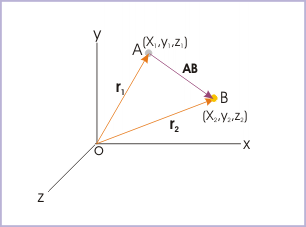# 1.12 Displacement

 Page 1 / 5
Motion involves two types of measurements : one which depends on the end points (displacement) and the other which depends on all points(distance) of motion.

Displacement is a measurement of change in position of the particle in motion. Its magnitude and direction are measured by the length and direction of the straight line joining initial and final positions of the particle. Obviously, the length of the straight line between the positions is the shortest distance between the points.

Displacement
Displacement is the vector extending from initial to final positions of the particle in motion during an interval.

From physical view point, displacement conveys the meaning of shortest distance plus direction of the motion between two time instants or corresponding two positions. Initial and final positions of the point object are the only important consideration for measuring magnitude of displacement. Actual path between two positions has no consequence in so far as displacement is concerned.

The quantum of displacement is measured by the length of the straight line joining two ends of motion. If there is no change in the position at the end of a motion, the displacement is zero.

In order to illustrate the underlying concept of displacement, let us consider the motion of a particle from A to B to C. The displacement vector is represented by the vector AC and its magnitude by the length of AC.

Once motion has begun, magnitude of displacement may increase or decrease (at a slow, fast or constant rate) or may even be zero, if the object returns to its initial position. Since a body under motion can take any arbitrary path, it is always possible that the end point of the motion may come closer or may go farther away from the initial point. Thus, displacement, unlike distance, may decrease from a given level.

In order to understand the variations in displacement with the progress of motion, let us consider another example of the motion of a particle along the rectangular path from A to B to C to D to A. Magnitude of displacement, shown by dotted vectors, is increasing during motion from A to B to C. Whereas magnitude of displacement is decreasing as the particle moves from C to D to A, eventually being equal to zero, when the particle returns to A.

However, displacement is essentially a measurement of length combined with direction. As direction has no dimension, its dimensional formula is also [L] like that of distance; and likewise, its SI measurement unit is ‘meter’.

## Displacement and position vector

We have the liberty to describe displacement vector as an independent vector ( AB ) or in terms of position vectors ( ${\mathbf{r}}_{1}$ and ${\mathbf{r}}_{2}$ ). The choice depends on the problem in hand. The description, however, is equivalent.

Let us consider that a point object moves from point A (represented by position vector ${\mathbf{r}}_{1}$ ) to point B (represented by position vector ${\mathbf{r}}_{2}$ ) as shown in the figure. Now, using triangle law (moving from O to A to B to O), we have :Displacement is equal to the difference between final and initial position vectors

what are scalars
show that 1w= 10^7ergs^-1
what's lamin's theorems and it's mathematics representative
if the wavelength is double,what is the frequency of the wave
What are the system of units
A stone propelled from a catapult with a speed of 50ms-1 attains a height of 100m. Calculate the time of flight, calculate the angle of projection, calculate the range attained
58asagravitasnal firce
Amar
water boil at 100 and why
what is upper limit of speed
what temperature is 0 k
Riya
0k is the lower limit of the themordynamic scale which is equalt to -273 In celcius scale
Mustapha
How MKS system is the subset of SI system?
which colour has the shortest wavelength in the white light spectrum
if x=a-b, a=5.8cm b=3.22 cm find percentage error in x
x=5.8-3.22 x=2.58
what is the definition of resolution of forces
what is energy?
Ability of doing work is called energy energy neither be create nor destryoed but change in one form to an other form
Abdul
motion
Mustapha
highlights of atomic physics
Benjamin
can anyone tell who founded equations of motion !?ByByBy